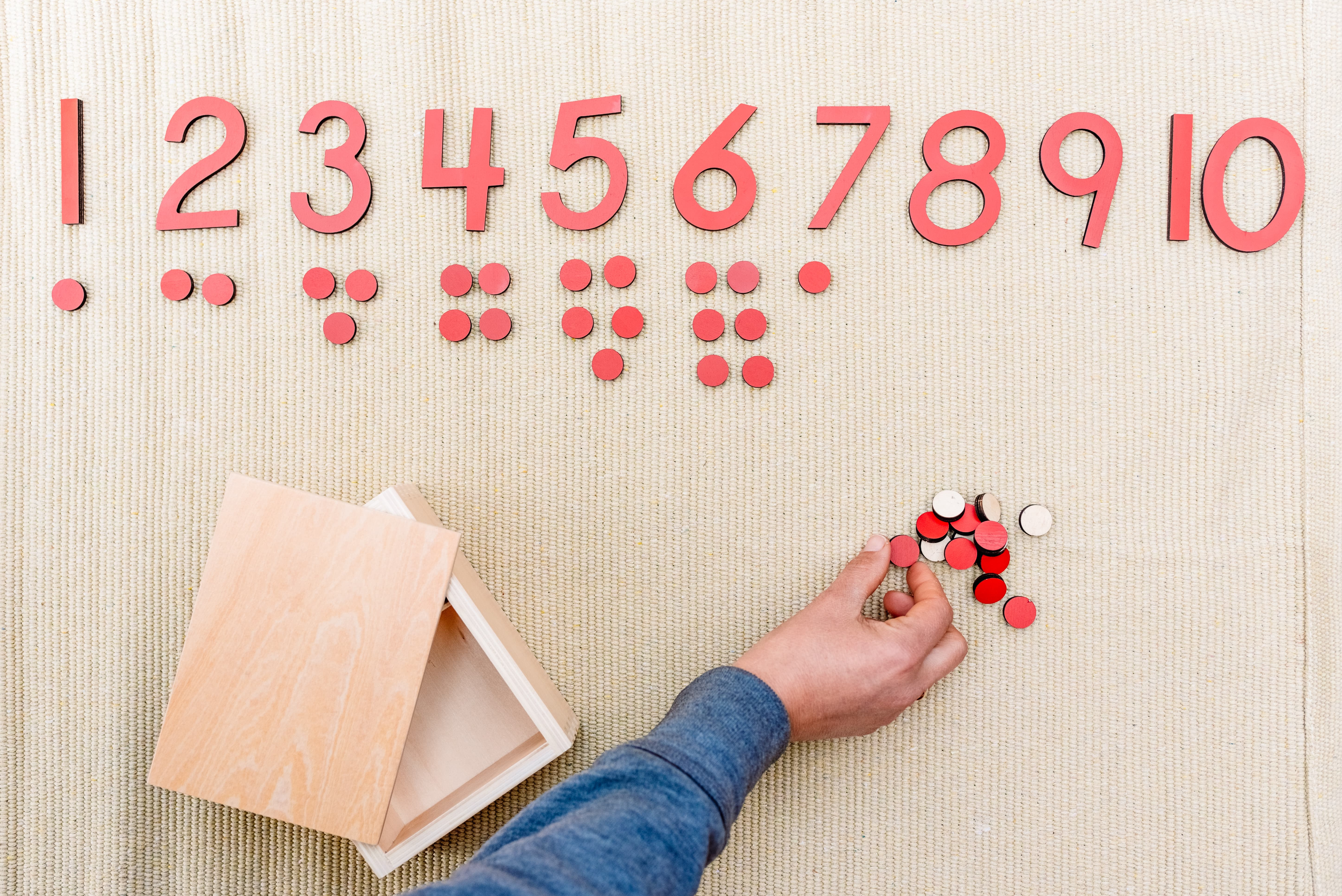Nirmala V Mani

## Montessori Materials Spotlight - Numerals and Counters

This exercise is designed to help children understand the connection between numerals and quantities, as well as the sequence of numbers. It also introduces the concept of odd and even numbers.

The purpose of this exercise is to help children understand the connection between numbers and quantities and to introduce the concept of odd and even numbers, making it a great foundation for future mathematical learning.

### Introducing the concept of Numerals and Counters:

The set includes ten numerals. Start with numbers 1 to 10 and wait until your child is comfortable with the sequence and quantities before continuing. The counters are arranged in twos, with a single counter at the bottom for odd numbers, so make sure to follow the setup.### Materials needed

• Numeral and Counter set
• Floor mat

The steps of the activity are as follows:

1. Invite your child to join you.
2. Let your child unroll the mat.
3. Bring the box of numerals and counters to the mat.
4. Line up the numbers 1 to 5, saying each one as you place it on the mat.
5. Place counters under each number, counting as you do it ("one, two, three..."), with the counters arranged in rows of two.
6. Put the counters back in the box.
7. Ask your child if they would like to put the counters under the numbers and let them do it.
8. If your child is working well with the material, introduce the rest of the numbers. Otherwise, leave them for another lesson.
9. Put everything away together.